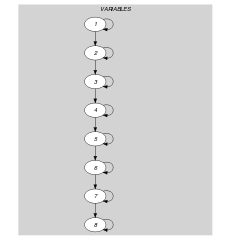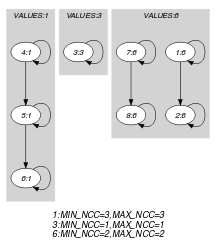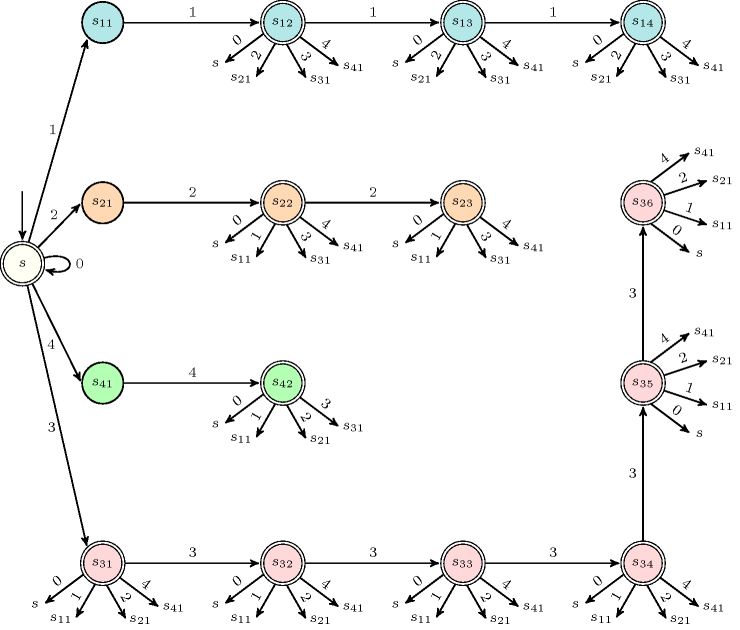## 5.376. stretch_path

Origin
Constraint

$\mathrm{𝚜𝚝𝚛𝚎𝚝𝚌𝚑}_\mathrm{𝚙𝚊𝚝𝚑}\left(\mathrm{𝚅𝙰𝚁𝙸𝙰𝙱𝙻𝙴𝚂},\mathrm{𝚅𝙰𝙻𝚄𝙴𝚂}\right)$

Usual name

$\mathrm{𝚜𝚝𝚛𝚎𝚝𝚌𝚑}$

Arguments
 $\mathrm{𝚅𝙰𝚁𝙸𝙰𝙱𝙻𝙴𝚂}$ $\mathrm{𝚌𝚘𝚕𝚕𝚎𝚌𝚝𝚒𝚘𝚗}\left(\mathrm{𝚟𝚊𝚛}-\mathrm{𝚍𝚟𝚊𝚛}\right)$ $\mathrm{𝚅𝙰𝙻𝚄𝙴𝚂}$ $\mathrm{𝚌𝚘𝚕𝚕𝚎𝚌𝚝𝚒𝚘𝚗}\left(\mathrm{𝚟𝚊𝚕}-\mathrm{𝚒𝚗𝚝},\mathrm{𝚕𝚖𝚒𝚗}-\mathrm{𝚒𝚗𝚝},\mathrm{𝚕𝚖𝚊𝚡}-\mathrm{𝚒𝚗𝚝}\right)$
Restrictions
 $|\mathrm{𝚅𝙰𝚁𝙸𝙰𝙱𝙻𝙴𝚂}|>0$ $\mathrm{𝚛𝚎𝚚𝚞𝚒𝚛𝚎𝚍}$$\left(\mathrm{𝚅𝙰𝚁𝙸𝙰𝙱𝙻𝙴𝚂},\mathrm{𝚟𝚊𝚛}\right)$ $|\mathrm{𝚅𝙰𝙻𝚄𝙴𝚂}|>0$ $\mathrm{𝚛𝚎𝚚𝚞𝚒𝚛𝚎𝚍}$$\left(\mathrm{𝚅𝙰𝙻𝚄𝙴𝚂},\left[\mathrm{𝚟𝚊𝚕},\mathrm{𝚕𝚖𝚒𝚗},\mathrm{𝚕𝚖𝚊𝚡}\right]\right)$ $\mathrm{𝚍𝚒𝚜𝚝𝚒𝚗𝚌𝚝}$$\left(\mathrm{𝚅𝙰𝙻𝚄𝙴𝚂},\mathrm{𝚟𝚊𝚕}\right)$ $\mathrm{𝚅𝙰𝙻𝚄𝙴𝚂}.\mathrm{𝚕𝚖𝚒𝚗}\ge 0$ $\mathrm{𝚅𝙰𝙻𝚄𝙴𝚂}.\mathrm{𝚕𝚖𝚒𝚗}\le \mathrm{𝚅𝙰𝙻𝚄𝙴𝚂}.\mathrm{𝚕𝚖𝚊𝚡}$ $\mathrm{𝚅𝙰𝙻𝚄𝙴𝚂}.\mathrm{𝚕𝚖𝚒𝚗}\le |\mathrm{𝚅𝙰𝚁𝙸𝙰𝙱𝙻𝙴𝚂}|$
Purpose

In order to define the meaning of the $\mathrm{𝚜𝚝𝚛𝚎𝚝𝚌𝚑}_\mathrm{𝚙𝚊𝚝𝚑}$ constraint, we first introduce the notions of stretch and span. Let $n$ be the number of variables of the collection $\mathrm{𝚅𝙰𝚁𝙸𝙰𝙱𝙻𝙴𝚂}$. Let ${X}_{i},\cdots ,{X}_{j}$ $\left(1\le i\le j\le n\right)$ be consecutive variables of the collection of variables $\mathrm{𝚅𝙰𝚁𝙸𝙰𝙱𝙻𝙴𝚂}$ such that the following conditions apply:

• All variables ${X}_{i},\cdots ,{X}_{j}$ take a same value from the set of values of the $\mathrm{𝚟𝚊𝚕}$ attribute,

• $i=1$ or ${X}_{i-1}$ is different from ${X}_{i}$,

• $j=n$ or ${X}_{j+1}$ is different from ${X}_{j}$.

We call such a set of variables a stretch. The span of the stretch is equal to $j-i+1$, while the value of the stretch is ${X}_{i}$. We now define the condition enforced by the $\mathrm{𝚜𝚝𝚛𝚎𝚝𝚌𝚑}_\mathrm{𝚙𝚊𝚝𝚑}$ constraint.

Each item $\left(\mathrm{𝚟𝚊𝚕}-v,\mathrm{𝚕𝚖𝚒𝚗}-s,\mathrm{𝚕𝚖𝚊𝚡}-t\right)$ of the $\mathrm{𝚅𝙰𝙻𝚄𝙴𝚂}$ collection enforces the minimum value $s$ as well as the maximum value $t$ for the span of a stretch of value $v$ over consecutive variables of the $\mathrm{𝚅𝙰𝚁𝙸𝙰𝙱𝙻𝙴𝚂}$ collection.

Note that:

1. Having an item $\left(\mathrm{𝚟𝚊𝚕}-v,\mathrm{𝚕𝚖𝚒𝚗}-s,\mathrm{𝚕𝚖𝚊𝚡}-t\right)$ with $s$ strictly greater than 0 does not mean that value $v$ should be assigned to one of the variables of collection $\mathrm{𝚅𝙰𝚁𝙸𝙰𝙱𝙻𝙴𝚂}$. It rather means that, when value $v$ is used, all stretches of value $v$ must have a span that belong to interval $\left[s,t\right]$.

2. A variable of the collection $\mathrm{𝚅𝙰𝚁𝙸𝙰𝙱𝙻𝙴𝚂}$ may be assigned a value that is not defined in the $\mathrm{𝚅𝙰𝙻𝚄𝙴𝚂}$ collection.

Example
$\left(\begin{array}{c}〈6,6,3,1,1,1,6,6〉,\hfill \\ 〈\begin{array}{ccc}\mathrm{𝚟𝚊𝚕}-1\hfill & \mathrm{𝚕𝚖𝚒𝚗}-2\hfill & \mathrm{𝚕𝚖𝚊𝚡}-4,\hfill \\ \mathrm{𝚟𝚊𝚕}-2\hfill & \mathrm{𝚕𝚖𝚒𝚗}-2\hfill & \mathrm{𝚕𝚖𝚊𝚡}-3,\hfill \\ \mathrm{𝚟𝚊𝚕}-3\hfill & \mathrm{𝚕𝚖𝚒𝚗}-1\hfill & \mathrm{𝚕𝚖𝚊𝚡}-6,\hfill \\ \mathrm{𝚟𝚊𝚕}-6\hfill & \mathrm{𝚕𝚖𝚒𝚗}-2\hfill & \mathrm{𝚕𝚖𝚊𝚡}-2\hfill \end{array}〉\hfill \end{array}\right)$

The $\mathrm{𝚜𝚝𝚛𝚎𝚝𝚌𝚑}_\mathrm{𝚙𝚊𝚝𝚑}$ constraint holds since the sequence $66311166$ contains four stretches $66$, 3, $111$, and $66$ respectively verifying the following conditions:

• The span of the first stretch $66$ is located within interval $\left[2,2\right]$ (i.e., the limit associated with value 6).

• The span of the second stretch 3 is located within interval $\left[1,6\right]$ (i.e., the limit associated with value 3).

• The span of the third stretch $111$ is located within interval $\left[2,4\right]$ (i.e., the limit associated with value 1).

• The span of the fourth stretch $66$ is located within interval $\left[2,2\right]$ (i.e., the limit associated with value 6).

Typical
 $|\mathrm{𝚅𝙰𝚁𝙸𝙰𝙱𝙻𝙴𝚂}|>1$ $\mathrm{𝚛𝚊𝚗𝚐𝚎}$$\left(\mathrm{𝚅𝙰𝚁𝙸𝙰𝙱𝙻𝙴𝚂}.\mathrm{𝚟𝚊𝚛}\right)>1$ $|\mathrm{𝚅𝙰𝚁𝙸𝙰𝙱𝙻𝙴𝚂}|>|\mathrm{𝚅𝙰𝙻𝚄𝙴𝚂}|$ $|\mathrm{𝚅𝙰𝙻𝚄𝙴𝚂}|>1$ $\mathrm{𝚜𝚞𝚖}$$\left(\mathrm{𝚅𝙰𝙻𝚄𝙴𝚂}.\mathrm{𝚕𝚖𝚒𝚗}\right)\le |\mathrm{𝚅𝙰𝚁𝙸𝙰𝙱𝙻𝙴𝚂}|$ $\mathrm{𝚅𝙰𝙻𝚄𝙴𝚂}.\mathrm{𝚕𝚖𝚊𝚡}\le |\mathrm{𝚅𝙰𝚁𝙸𝙰𝙱𝙻𝙴𝚂}|$
Symmetries
• Items of $\mathrm{𝚅𝙰𝚁𝙸𝙰𝙱𝙻𝙴𝚂}$ can be reversed.

• Items of $\mathrm{𝚅𝙰𝙻𝚄𝙴𝚂}$ are permutable.

• All occurrences of two distinct values in $\mathrm{𝚅𝙰𝚁𝙸𝙰𝙱𝙻𝙴𝚂}.\mathrm{𝚟𝚊𝚛}$ or $\mathrm{𝚅𝙰𝙻𝚄𝙴𝚂}.\mathrm{𝚟𝚊𝚕}$ can be swapped; all occurrences of a value in $\mathrm{𝚅𝙰𝚁𝙸𝙰𝙱𝙻𝙴𝚂}.\mathrm{𝚟𝚊𝚛}$ or $\mathrm{𝚅𝙰𝙻𝚄𝙴𝚂}.\mathrm{𝚟𝚊𝚕}$ can be renamed to any unused value.

Usage

The article [Pesant01], which originally introduced the $\mathrm{𝚜𝚝𝚛𝚎𝚝𝚌𝚑}$ constraint, quotes rostering problems as typical examples of use of this constraint.

Remark

We split the original $\mathrm{𝚜𝚝𝚛𝚎𝚝𝚌𝚑}$ constraint into the $\mathrm{𝚜𝚝𝚛𝚎𝚝𝚌𝚑}_\mathrm{𝚙𝚊𝚝𝚑}$ and the $\mathrm{𝚜𝚝𝚛𝚎𝚝𝚌𝚑}_\mathrm{𝚌𝚒𝚛𝚌𝚞𝚒𝚝}$ constraints that respectively use the $\mathrm{𝑃𝐴𝑇𝐻}$ $\mathrm{𝐿𝑂𝑂𝑃}$ and the $\mathrm{𝐶𝐼𝑅𝐶𝑈𝐼𝑇}$ $\mathrm{𝐿𝑂𝑂𝑃}$ arc generators. We also reorganise the parameters: the $\mathrm{𝚅𝙰𝙻𝚄𝙴𝚂}$ collection describes the attributes of each value that can be assigned to the variables of the $\mathrm{𝚜𝚝𝚛𝚎𝚝𝚌𝚑}_\mathrm{𝚙𝚊𝚝𝚑}$ constraint. Finally we skipped the pattern constraint that tells what values can follow a given value. A extension of this constraint (i.e., stretch plus pattern), called $\mathrm{𝚏𝚘𝚛𝚌𝚎𝚍}_\mathrm{𝚜𝚑𝚒𝚏𝚝}_\mathrm{𝚜𝚝𝚛𝚎𝚝𝚌𝚑}$, where one can specify for each value $v$ with a 0-1 variable, whether it should occur at least once or not at all, was proposed in [HellstenPesantBeek04]. By reduction to Hamiltonian path it was shown that enforcing arc-consistency for $\mathrm{𝚏𝚘𝚛𝚌𝚎𝚍}_\mathrm{𝚜𝚑𝚒𝚏𝚝}_\mathrm{𝚜𝚝𝚛𝚎𝚝𝚌𝚑}$ is NP-hard [HellstenPesantBeek04].

Algorithm

A first filtering algorithm was described in the original article of G. Pesant [Pesant01]. A second filtering algorithm, based on dynamic programming, achieving arc-consistency is depicted in  [Hellsten04], [HellstenPesantBeek04]. It also handles the fact that some values can be followed by only a given subset of values. An other alternative achieving arc-consistency is to use the automaton described in the Automaton slot.

Systems

generalisation: $\mathrm{𝚜𝚝𝚛𝚎𝚝𝚌𝚑}_\mathrm{𝚙𝚊𝚝𝚑}_\mathrm{𝚙𝚊𝚛𝚝𝚒𝚝𝚒𝚘𝚗}$ ($\mathrm{𝚟𝚊𝚛𝚒𝚊𝚋𝚕𝚎}$ replaced by $\mathrm{𝚟𝚊𝚛𝚒𝚊𝚋𝚕𝚎}\in \mathrm{𝚙𝚊𝚛𝚝𝚒𝚝𝚒𝚘𝚗}$).

Keywords

For all items of $\mathrm{𝚅𝙰𝙻𝚄𝙴𝚂}$:

Arc input(s)

$\mathrm{𝚅𝙰𝚁𝙸𝙰𝙱𝙻𝙴𝚂}$

Arc generator
 $\mathrm{𝑃𝐴𝑇𝐻}$$↦\mathrm{𝚌𝚘𝚕𝚕𝚎𝚌𝚝𝚒𝚘𝚗}\left(\mathrm{𝚟𝚊𝚛𝚒𝚊𝚋𝚕𝚎𝚜}\mathtt{1},\mathrm{𝚟𝚊𝚛𝚒𝚊𝚋𝚕𝚎𝚜}\mathtt{2}\right)$ $\mathrm{𝐿𝑂𝑂𝑃}$$↦\mathrm{𝚌𝚘𝚕𝚕𝚎𝚌𝚝𝚒𝚘𝚗}\left(\mathrm{𝚟𝚊𝚛𝚒𝚊𝚋𝚕𝚎𝚜}\mathtt{1},\mathrm{𝚟𝚊𝚛𝚒𝚊𝚋𝚕𝚎𝚜}\mathtt{2}\right)$

Arc arity
Arc constraint(s)
 $•\mathrm{𝚟𝚊𝚛𝚒𝚊𝚋𝚕𝚎𝚜}\mathtt{1}.\mathrm{𝚟𝚊𝚛}=\mathrm{𝚅𝙰𝙻𝚄𝙴𝚂}.\mathrm{𝚟𝚊𝚕}$ $•\mathrm{𝚟𝚊𝚛𝚒𝚊𝚋𝚕𝚎𝚜}\mathtt{2}.\mathrm{𝚟𝚊𝚛}=\mathrm{𝚅𝙰𝙻𝚄𝙴𝚂}.\mathrm{𝚟𝚊𝚕}$
Graph property(ies)
 $•$$\mathrm{𝚗𝚘𝚝}_\mathrm{𝚒𝚗}$$\left($$\mathrm{𝐌𝐈𝐍}_\mathrm{𝐍𝐂𝐂}$$,1,\mathrm{𝚅𝙰𝙻𝚄𝙴𝚂}.\mathrm{𝚕𝚖𝚒𝚗}-1\right)$ $•$$\mathrm{𝐌𝐀𝐗}_\mathrm{𝐍𝐂𝐂}$$\le \mathrm{𝚅𝙰𝙻𝚄𝙴𝚂}.\mathrm{𝚕𝚖𝚊𝚡}$

Graph model

Part (A) of Figure 5.376.1 shows the initial graphs associated with values 1, 2, 3 and 6 of the Example slot. Part (B) of Figure 5.376.1 shows the corresponding final graphs associated with values 1, 3 and 6. Since value 2 is not assigned to any variable of the $\mathrm{𝚅𝙰𝚁𝙸𝙰𝙱𝙻𝙴𝚂}$ collection the final graph associated with value 2 is empty. The $\mathrm{𝚜𝚝𝚛𝚎𝚝𝚌𝚑}_\mathrm{𝚙𝚊𝚝𝚑}$ constraint holds since:

• For value 1 we have one connected component for which the number of vertices 3 is greater than or equal to 2 and less than or equal to 4,

• For value 2 we do not have any connected component,

• For value 3 we have one connected component for which the number of vertices 1 is greater than or equal to 1 and less than or equal to 6,

• For value 6 we have two connected components that both contain two vertices: this is greater than or equal to 2 and less than or equal to 2.

##### Figure 5.376.1. Initial and final graph of the $\mathrm{𝚜𝚝𝚛𝚎𝚝𝚌𝚑}_\mathrm{𝚙𝚊𝚝𝚑}$ constraint(a) (b)

During the presentation of this constraint at CP'2001 the following point was mentioned: it could be useful to allow domain variables for the minimum and the maximum values of a stretch. This could be achieved in the following way: the $\mathrm{𝚕𝚖𝚒𝚗}$ (respectively $\mathrm{𝚕𝚖𝚊𝚡}$) attribute would now be a domain variable that gives the size of the shortest (respectively longest) stretch. Finally within the Graph property(ies) slot we would replace $\ge$ (and $\le$) by $=$.

Automaton

Let $n$ and $m$ respectively denote the quantities $|\mathrm{𝚅𝙰𝚁𝙸𝙰𝙱𝙻𝙴𝚂}|$ and $|\mathrm{𝚅𝙰𝙻𝚄𝙴𝚂}|$. Furthermore, let ${\mathrm{𝚟𝚊𝚕}}_{i}$, ${\mathrm{𝚕𝚖𝚒𝚗}}_{i}$ and ${\mathrm{𝚕𝚖𝚊𝚡}}_{i}$, $\left(i\in \left[1,m\right]\right)$, respectively be shortcuts for the expressions $\mathrm{𝚅𝙰𝙻𝚄𝙴𝚂}\left[i\right].\mathrm{𝚟𝚊𝚕}$, $\mathrm{𝚅𝙰𝙻𝚄𝙴𝚂}\left[i\right].\mathrm{𝚕𝚖𝚒𝚗}$ and $\mathrm{𝚅𝙰𝙻𝚄𝙴𝚂}\left[i\right].\mathrm{𝚕𝚖𝚊𝚡}$. Without loss of generality, we assume that all the $\mathrm{𝚕𝚖𝚒𝚗}$ attributes of the items of the $\mathrm{𝚅𝙰𝙻𝚄𝙴𝚂}$ collection are at least equal to 1. The following automaton $𝒜$ involving $1+{\mathrm{𝚕𝚖𝚊𝚡}}_{1}+{\mathrm{𝚕𝚖𝚊𝚡}}_{2}+\cdots +{\mathrm{𝚕𝚖𝚊𝚡}}_{m}$ states only accepts solutions to the $\mathrm{𝚜𝚝𝚛𝚎𝚝𝚌𝚑}_\mathrm{𝚙𝚊𝚝𝚑}$ constraint. Automaton $𝒜$ has the following states:

• an initial state $s$ that is also an accepting state,

• $\forall i\in \left[1,m\right],\forall j\in \left[1,{\mathrm{𝚕𝚖𝚒𝚗}}_{i}-1\right]$, a non-accepting state ${s}_{i,j}$,

• $\forall i\in \left[1,m\right],\forall j\in \left[{\mathrm{𝚕𝚖𝚒𝚗}}_{i},{\mathrm{𝚕𝚖𝚊𝚡}}_{i}\right]$, an accepting state ${s}_{i,j}$.

Transitions of $𝒜$ are defined in the following way:

• $\forall i\in \left[1,m\right]$, a transition from $s$ to ${s}_{i,1}$ labelled by condition ${X}_{l}={\mathrm{𝚟𝚊𝚕}}_{i}$,

• a transition from $s$ to $s$ labelled by condition ${X}_{l}\ne {\mathrm{𝚟𝚊𝚕}}_{1}\wedge {X}_{l}\ne {\mathrm{𝚟𝚊𝚕}}_{2}\wedge \cdots \wedge {X}_{l}\ne {\mathrm{𝚟𝚊𝚕}}_{m}$,

• $\forall i\in \left[1,m\right],\forall j\in \left[{\mathrm{𝚕𝚖𝚒𝚗}}_{i},{\mathrm{𝚕𝚖𝚊𝚡}}_{i}\right]$, a transition from ${s}_{i,j}$ to $s$ labelled by condition ${X}_{l}\ne {\mathrm{𝚟𝚊𝚕}}_{1}\wedge {X}_{l}\ne {\mathrm{𝚟𝚊𝚕}}_{2}\wedge \cdots \wedge {X}_{l}\ne {\mathrm{𝚟𝚊𝚕}}_{m}$,

• $\forall i\in \left[1,m\right],\forall j\in \left[1,{\mathrm{𝚕𝚖𝚊𝚡}}_{i}-1\right]$, a transition from ${s}_{i,j}$ to ${s}_{i,j+1}$ labelled by condition ${X}_{l}={\mathrm{𝚟𝚊𝚕}}_{i}$,

• $\forall i\in \left[1,m\right],\forall j\in \left[{\mathrm{𝚕𝚖𝚒𝚗}}_{i},{\mathrm{𝚕𝚖𝚊𝚡}}_{i}\right],\forall k\ne i\in \left[1,m\right]$, a transition from ${s}_{i,j}$ to ${s}_{k,1}$ labelled by condition ${X}_{l}={\mathrm{𝚟𝚊𝚕}}_{k}$.

Figure 5.376.2 depicts the automaton associated with the $\mathrm{𝚜𝚝𝚛𝚎𝚝𝚌𝚑}_\mathrm{𝚙𝚊𝚝𝚑}$ constraint of the Example slot. Transitions labels 0, 1, 2, 3 and 4 respectively correspond to the conditions ${X}_{l}\ne 1\wedge {X}_{l}\ne 2\wedge {X}_{l}\ne 3\wedge {X}_{l}\ne 6$, ${X}_{l}=1$, ${X}_{l}=2$, ${X}_{l}=3$, ${X}_{l}=6$ (since values 1, 2, 3 and 6 respectively correspond to the values of the first, second, third and fourth item of the $\mathrm{𝚅𝙰𝙻𝚄𝙴𝚂}$ collection). The $\mathrm{𝚜𝚝𝚛𝚎𝚝𝚌𝚑}_\mathrm{𝚙𝚊𝚝𝚑}$ constraint holds since the corresponding sequence of visited states, $s$ ${s}_{41}$ ${s}_{42}$ ${s}_{31}$ ${s}_{11}$ ${s}_{12}$ ${s}_{13}$ ${s}_{41}$ ${s}_{42}$, ends up in an accepting state (i.e., accepting states are denoted graphically by a double circle in the figure).

##### Figure 5.376.2. Automaton of the $\mathrm{𝚜𝚝𝚛𝚎𝚝𝚌𝚑}_\mathrm{𝚙𝚊𝚝𝚑}$ constraint of the Example slot (states related to a same stretch have the same colour)